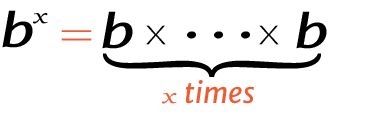# 6 raise to the power of 2

Place the base and exponent in the power calculator to get the right answer for value of 2^6, 26 (2 power 6), or 6 raised to the power of 2.

## How to calculate 2 raise to the power of 6?

This is how you can solve (2) 6 manually.

Solution:

Step 1: Simplify the data.

Base
ExponentStep 2: Multiply the 2 to itself 6 times.

Using this exponents calculator could be much easier to figure out an exponential expression.